• ## 均方误差

万次阅读 2018-09-24 17:27:55
均方误差 一、均方误差的含义及公式 均方误差是衡量“平均误差”的一种较方便的方法，可以评价数据的变化程度。从类别来看属于预测评价与预测组合；从字面上看来，“均”指的是平均，即求其平均值，“方差”即是在...
均方误差
一、均方误差的含义及公式
均方误差是衡量“平均误差”的一种较方便的方法，可以评价数据的变化程度。从类别来看属于预测评价与预测组合；从字面上看来，“均”指的是平均，即求其平均值，“方差”即是在概率论中用来衡量随机变量和其估计值（其平均值）之间的偏离程度的度量值，“误”可以理解为测定值与真实值之间的误差。 均方误差的公式：MSE =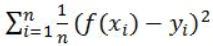。
二、均方误差的推导
均方误差可以通过平均误差来推导： 平方误差：表示实验误差大小的偏差平方和。在相同的条件下，各次测定值xi对真实值x的偏差平方后再求和，即：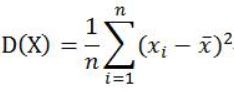均方误差是各数据偏离真实值的距离平方和的平均数，所以用真实值减去其的误差的估计值平方和求其平均数，即：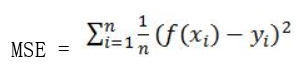估计值就是通过对之前的各个条件的x值和y值拟合出一个公式，通过公式，将新得到的x值带入求出一个y的估计值，与此同时会有一个y的真实值，再将y的估计值和真实值带入均方误差的公式中，求出一个均方误差的值，如果均方误差的值越小则说明模型的拟合实验数据能力强，但不能使它等于0，当其为0的时候则说明了这个模型完全拟合我们列出的条件，但可能现实中有我们没有考虑到的部分， 可能会使模型出现大波动，所以当均方误差值为0时，这个模型是不适用的，我们最好不要使用这个模型。
三、均方误差和均方根误差以及平均绝对误差之间的区别
为了更好的了解均方误差，我们必须要对均方误差相近的知识了解清楚，那么我们今天就来了解一下均方误差(MSE)和均方根误差(RMSE)和平均绝对误差(MAE)分别是什么： （1）均方误差（Mean Squared Error）: 均方误差是反映估计量与被估计量之间差异程度的一种度量。设t是根据子样确定的总体参数θ的一个估计量，(θ-t)2的数学期望，称为估计量t的均方误差。它等于σ2+b2，其中σ2与b分别是t的方差与偏倚。均方误差是指参数估计值与参数真值之差平方的期望值; 它可以评价数据的变化程度，当MSE的值越小时也就说明预测模型描述实验数据具有更好的精确度。 （2）均方根误差（Root Mean Square Error）：均方根误差亦称标准误差，其定义为 ，i=1，2，3，…n。在有限测量次数中，均方根误差常用下式表示：√[∑di2/n]=Re，式中：n为测量次数；di为一组测量值与真值的偏差。在有限测量次数中，均方根误差常用下式表示：√[∑di2/n]=Re，式中:n为测量次数；di为一组测量值与真值的偏差。如果误差统计分布是正态分布，那么随机误差落在±σ以内的概率为68%。均方根误差是均方误差的算术平方根。 （3）平均绝对误差（Mean Absolute Error）：又叫平均绝对离差，是所有单个观测值与算术平均值的偏差的绝对值的平均。平均绝对误差可以避免误差相互抵消的问题，因而可以准确反映实际预测误差的大小。平均绝对误差是绝对误差的平均值，平均绝对误差能更好地反映预测值误差的实际情况。
四、总结
通过这次对均方误差的学习，我们了解到了什么是均方误差、均方误差的推导是如何实现的、以及均方误差、均方根误差和平均绝对误差的区别，我们知道了如何运用均方误差来衡量一个模型是否能够较强的拟合实验数据，在以后的实践中，我们争取运用均方误差来衡量我们构建的模型是否能够实现我们想要达到的预期效果。
如果有误，望各位大神指正！
展开全文• 方差、协方差、标准差(标准偏差/均方差）、均方误差、均方根误差(标准误差)、均方根值 本文由博主经过查阅网上资料整理总结后编写，如存在错误或不恰当之处请留言以便更正，内容仅供大家参考学习。 方差...
  方差、协方差、标准差(标准偏差/均方差）、均方误差、均方根误差(标准误差)、均方根值
本文由博主经过查阅网上资料整理总结后编写，如存在错误或不恰当之处请留言以便更正，内容仅供大家参考学习。

方差（Variance)
方差用于衡量随机变量或一组数据的离散程度，方差在在统计描述和概率分布中有不同的定义和计算公式。①概率论中方差用来度量随机变量和其数学期望（即均值）之间的偏离程度；②统计中的方差(样本方差)是每个样本值与全体样本均值之差的平方值的平均数，代表每个变量与总体均值间的离散程度。
概率论中计算公式
离散型随机变量的数学期望：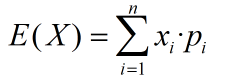---------求取期望值
连续型随机变量的数学期望：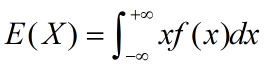----------求取期望值
其中，pi是变量，xi发生的概率，f(x)是概率密度。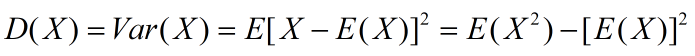---------求取方差值

统计学中计算公式
总体方差，也叫做有偏估计，其实就是我们从初高中就学到的那个标准定义的方差：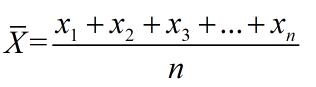-----------求取总体均值
其中，n表示这组数据个数，x1、x2、x3……xn表示这组数据具体数值。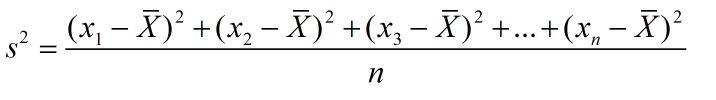------------求取总体方差
其中，为数据的平均数，n为数据的个数，为方差。
样本方差，无偏方差，在实际情况中，总体均值是很难得到的，往往通过抽样来计算，于是有样本方差，计算公式如下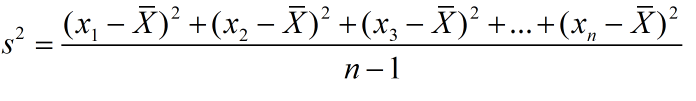--------------求取样本方差
此处，为什么要将分母由n变成n-1，主要是为了实现无偏估计减小误差，请阅读《为什么样本方差的分母是 n-1》。
协方差（Covariance）
协方差在概率论和统计学中用于衡量两个变量的总体误差。而方差是协方差的一种特殊情况，即当两个变量是相同的情况。协方差表示的是两个变量的总体的误差，这与只表示一个变量误差的方差不同。 如果两个变量的变化趋势一致，也就是说如果其中一个大于自身的期望值，另外一个也大于自身的期望值，那么两个变量之间的协方差就是正值。 如果两个变量的变化趋势相反，即其中一个大于自身的期望值，另外一个却小于自身的期望值，那么两个变量之间的协方差就是负值。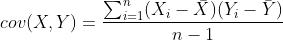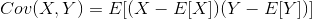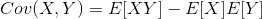其中，E[X]与E[Y]分别为两个实数随机变量X与Y的数学期望，Cov(X,Y)为X，Y的协方差。
标准差（Standard Deviation)
标准差也被称为标准偏差,在中文环境中又常称均方差，是数据偏离均值的平方和平均后的方根，用σ表示。标准差是方差的算术平方根。标准差能反映一个数据集的离散程度，只是由于方差出现了平方项造成量纲的倍数变化，无法直观反映出偏离程度，于是出现了标准差，标准偏差越小，这些值偏离平均值就越少，反之亦然。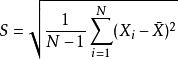------------求取样本标准差
其中，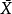代表所采用的样本X1,X2,...,Xn的均值。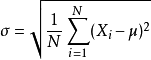-------------求取总体标准差
其中，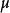代表总体X的均值。
例：有一组数字分别是200、50、100、200，求它们的样本标准偏差。= (200+50+100+200)/4 = 550/4 = 137.5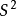= [(200-137.5)^2+(50-137.5)^2+(100-137.5)^2+(200-137.5)^2]/(4-1)
样本标准偏差 S = Sqrt(S^2)=75
均方误差（mean-square error, MSE）
均方误差是反映估计量与被估计量之间差异程度的一种度量，换句话说，参数估计值与参数真值之差的平方的期望值。MSE可以评价数据的变化程度，MSE的值越小，说明预测模型描述实验数据具有更好的精确度。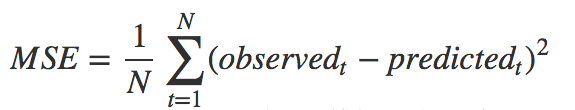均方根误差（root mean squared error，RMSE）
均方根误差亦称标准误差，是均方误差的算术平方根。换句话说，是观测值与真值(或模拟值)偏差(而不是观测值与其平均值之间的偏差)的平方与观测次数n比值的平方根，在实际测量中，观测次数n总是有限的，真值只能用最可信赖（最佳）值来代替。标准误差对一组测量中的特大或特小误差反映非常敏感，所以，标准误差能够很好地反映出测量的精密度。这正是标准误差在工程测量中广泛被采用的原因。因此，标准差是用来衡量一组数自身的离散程度，而均方根误差是用来衡量观测值同真值之间的偏差。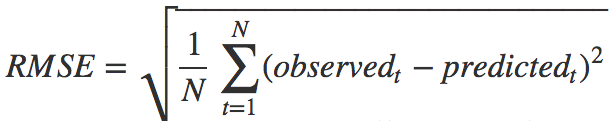均方根值（root-mean-square，RMES）
均方根值也称作为方均根值或有效值，在数据统计分析中，将所有值平方求和，求其均值，再开平方，就得到均方根值。在物理学中，我们常用均方根值来分析噪声。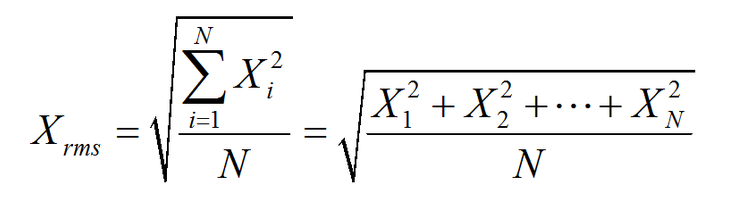比如幅度为100V而占空比为0.5的方波信号，如果按平均值计算，它的电压只有50V，而按均方根值计算则有70.71V。这是为什么呢？举一个例子，有一组100伏的电池组，每次供电10分钟之后停10分钟，也就是说占空比为一半。如果这组电池带动的是10Ω电阻，供电的10分钟产生10A 的电流和1000W的功率，停电时电流和功率为零。
展开全文• 评估图像的归一化均方误差 (NMRS) 作为滤波过程中去噪有效性和图像结构/细节保留的度量。 NMSE 表示过滤后的图像与真实图像的相似程度（在这种情况下，NMSE = 0）。matlab
• 这个 m 文件计算两个图像 x 和 y 之间的均方误差matlab
• 如果允许在强度上线性缩放一幅图像，则 LMSE 计算可能的最小均方误差 (MSE)。 LMSEDIFF 根据最小化 MSE 的缩放比例计算目标图像缩放后的差异图像。 如果强度的绝对幅度没有意义，这是比较图像的更好方法。matlab
• ## 均方误差(MSE)

万次阅读 多人点赞 2019-08-18 13:51:30
均方误差单独扽概念是很简单的，这里只做介绍，更深一步的内容会在后面列出来。 SSE(和方差、误差平方和)：The sum of squares due to error MSE(均方差、方差)：Mean squared error RMSE(均方根、标准差)：Root ...
均方误差单独扽概念是很简单的，这里只做介绍，更深一步的内容会在后面列出来。
SSE(和方差、误差平方和)：The sum of squares due to errorMSE(均方差、方差)：Mean squared errorRMSE(均方根、标准差)：Root mean squared error

数理统计中均方误差是指参数估计值与参数真值之差平方的期望值，记为MSE。MSE是衡量“平均误差”的一种较方便的方法，MSE可以评价数据的变化程度，MSE的值越小，说明预测模型描述实验数据具有更好的精确度。  首先先回顾复习三个概念：  1）方差：方差是在概率论和统计方差衡量随机变量或一组数据的离散程度的度量方式，方差越大，离散度越大。求解方式为，各随机变量与平均值差值的平方和的平均数（先求差，再平方，再平均）
平均数：

M

=

x

1

+

x

2

+

⋯

+

x

n

n

M=\frac{x_1+x_2+\cdots+x_n}{n}

方差公式：

s

2

=

(

x

1

−

M

)

2

+

(

x

1

−

M

)

2

+

⋯

+

(

x

n

−

M

)

2

n

s^2=\frac{(x_1-M)^2+(x_1-M)^2+\cdots+(x_n-M)^2}{n}

也可以通过以下的方式进行求解方差

D

(

x

)

=

E

(

x

2

)

−

(

E

(

x

)

)

2

D(x)=E(x^2)-(E(x))^2

2）标准差：标准差就是方差的算术平方根，它反映组内个体间的离散程度。因此它的过程是与平均值之间进行差值计算。

标准差公式：

σ

=

1

n

∑

i

=

1

n

(

x

i

−

μ

)

2

\sigma=\sqrt{\frac{1}{n}\sum_{i=1}^n(x_i-\mu)^2}

3）样本方差

σ

^

2

=

1

n

−

1

∑

i

=

1

n

(

x

i

−

μ

)

2

\hat{\sigma}^2=\frac{1}{n-1}\sum_{i=1}^n(x_i-\mu)^2

这里之所以列出样本方差的样子，是因为样本方差更多被采用，因为他是无偏估计的，只做了解。感兴趣的可以到网上搜下与方差的“无偏”证明。

1.SSE（和方差）
在统计学里，该参数计算的是拟合数据和原始数据对应点的误差的平方和，计算公式为，

S

S

E

=

∑

i

=

1

m

w

i

(

y

i

−

y

i

^

)

2

SSE=\sum_{i=1}^{m}w_i(y_i-\hat{y_i })^2

其中

y

i

y_i

是真实数据

y

i

^

\hat{y_i}

是拟合的数据，

w

i

>

0

w_i>0

从这里可以看出SSE越接近于0，说明模型选择和拟合更好，数据预测也越成功。接下来的MSE和RMSE因为和SSE是同出一宗，所以效果一样。

2.MSE（均方误差）
该统计参数是预测数据和原始数据对应点误差的平方和的均值，也就是

S

S

E

n

\frac{SSE}{n}

和

S

S

E

SSE

没有太大的区别，计算公式为:

M

S

E

=

S

S

E

n

=

1

n

∑

i

=

1

m

w

i

(

y

i

−

y

i

^

)

2

MSE=\frac{SSE}{n}=\frac{1}{n}\sum_{i=1}^{m}w_i(y_i-\hat{y_i })^2

其中

n

n

为样本的个数。

3.RMSE（均方根误差）
该统计参数，也叫回归系统的拟合标准差，是MSE的平方根，计算公式为

R

M

S

E

=

M

S

E

=

S

S

E

n

=

1

n

∑

i

=

1

m

w

i

(

y

i

−

y

i

^

)

2

RMSE=\sqrt{MSE}=\sqrt{\frac{SSE}{n}}=\sqrt{\frac{1}{n}\sum_{i=1}^{m}w_i(y_i-\hat{y_i })^2}

以上三个统计参数，虽然略有细微的差别，但是代表的都是数据拟合的好坏，只是标准不一样。

展开全文• 机器学习均方误差by Moshe Binieli 由Moshe Binieli 机器学习：均方误差和回归线简介 (Machine learning: an introduction to mean squared error and regression lines) 介绍 (Introduction) This article will ...

机器学习均方误差
by Moshe Binieli
由Moshe Binieli
机器学习：均方误差和回归线简介 (Machine learning: an introduction to mean squared error and regression lines)
介绍 (Introduction)
This article will deal with the statistical method mean squared error, and I’ll describe the relationship of this method to the regression line.
本文将讨论统计方法的均方误差 ，并描述该方法与回归线的关系。
The example consists of points on the Cartesian axis. We will define a mathematical function that will give us the straight line that passes best between all points on the Cartesian axis.
该示例由笛卡尔轴上的点组成。 我们将定义一个数学函数，该函数将为我们提供在笛卡尔轴上所有点之间最佳通过的直线。
And in this way, we will learn the connection between these two methods, and how the result of their connection looks together.
这样，我们将学习这两种方法之间的联系，以及它们的联系结果如何看待。
一般说明 (General explanation)
This is the definition from Wikipedia:
这是维基百科的定义：

In statistics, the mean squared error (MSE) of an estimator (of a procedure for estimating an unobserved quantity) measures the average of the squares of the errors — that is, the average squared difference between the estimated values and what is estimated. MSE is a risk function, corresponding to the expected value of the squared error loss. The fact that MSE is almost always strictly positive (and not zero) is because of randomness or because the estimator does not account for information that could produce a more accurate estimate.

在统计中，估计器(用于估计未观察到的数量的过程的估计器)的均方误差(MSE)测量误差平方的平均值，即，估计值与估计值之间的均方差。 MSE是一个风险函数，对应于平方误差损失的期望值。 MSE几乎始终严格为正数(而不是零)的事实是由于随机性，或者是因为估算器没有考虑可能产生更准确估算的信息。

文章的结构 (The structure of the article)
Get a feel for the idea, graph visualization, mean squared error equation. 了解一下这个想法，图形可视化，均方误差方程。 The mathematical part which contains algebraic manipulations and a derivative of two-variable functions for finding a minimum. This section is for those who want to understand how we get the mathematical formulas later, you can skip it if that doesn’t interest you. 数学部分，其中包含代数操作和用于寻找最小值的二变量函数的导数。 本节适用于那些想了解我们以后如何获得数学公式的人，如果您不感兴趣，可以跳过它。 An explanation of the mathematical formulae we received and the role of each variable in the formula. 对我们收到的数学公式的解释，以及每个变量在公式中的作用。 Examples 例子
感受一下这个主意 (Get a feel for the idea)
Let’s say we have seven points, and our goal is to find a line that minimizes the squared distances to these different points.
假设我们有七个点，我们的目标是找到一条线，以最小化到这些不同点的平方距离。
Let’s try to understand that.
让我们尝试了解一下。
I will take an example and I will draw a line between the points. Of course, my drawing isn’t the best, but it’s just for demonstration purposes.
我将举一个例子，并在两点之间画一条线。 当然，我的绘画不是最好的，但这只是出于演示目的。
You might be asking yourself, what is this graph?
您可能会问自己，这张图是什么？
the purple dots are the points on the graph. Each point has an x-coordinate and a y-coordinate. 紫色点是图形上的点。 每个点都有一个x坐标和一个y坐标。 The blue line is our prediction line. This is a line that passes through all the points and fits them in the best way. This line contains the predicted points. 蓝线是我们的预测线。 这是一条穿过所有点并以最佳方式拟合它们的线。 该行包含预测点。 The red line between each purple point and the prediction line are the errors. Each error is the distance from the point to its predicted point. 每个紫色点和预测线之间的红线是错误。 每个误差是从点到其预测点的距离。
You should remember this equation from your school days, y=Mx+B, where M is the slope of the line and B is y-intercept of the line.
您应该记得上学时的方程， y = Mx + B ，其中M是直线的斜率 ， B是直线的y截距 。
We want to find M (slope) and B (y-intercept) that minimizes the squared error!
我们想要找到最小化平方误差的M( 斜率 )和B( y截距 )！
Let’s define a mathematical equation that will give us the mean squared error for all our points.
让我们定义一个数学方程式，它将为我们提供所有点的均方误差。
Let’s analyze what this equation actually means.
让我们分析一下这个方程的实际含义。
In mathematics, the character that looks like weird E is called summation (Greek sigma). It is the sum of a sequence of numbers, from i=1 to n. Let’s imagine this like an array of points, where we go through all the points, from the first (i=1) to the last (i=n). 在数学中，看起来像奇怪的E的字符称为求和(Greek sigma)。 它是从i = 1到n的数字序列的总和。 让我们想象这就像一个点数组，我们从头一个点(i = 1)到最后一个点(i = n)遍历所有点。 For each point, we take the y-coordinate of the point, and the y’-coordinate. The y-coordinate is our purple dot. The y’ point sits on the line we created. We subtract the y-coordinate value from the y’-coordinate value, and calculate the square of the result. 对于每个点，我们采用该点的y坐标和y'坐标。 y坐标是我们的紫色圆点。 y'点位于我们创建的直线上。 我们从y'坐标值中减去y坐标值，然后计算结果的平方。 The third part is to take the sum of all the (y-y’)² values, and divide it by n, which will give the mean. 第三部分是取所有(y-y')²值的总和，然后除以n，得到平均值。
Our goal is to minimize this mean, which will provide us with the best line that goes through all the points.
我们的目标是尽量减少这种均值，这将为我们提供贯穿所有要点的最佳路线。
从概念到数学方程 (From concept to mathematical equations)
This part is for people who want to understand how we got to the mathematical equations. You can skip to the next part if you want.
这部分适用于那些想了解我们如何掌握数学方程的人们 。 您可以根据需要跳到下一部分。
As you know, the line equation is y=mx+b, where m is the slope and b is the y-intercept.
如您所知，线方程为y = mx + b，其中m为斜率 ，b为y截距 。
Let’s take each point on the graph, and we’ll do our calculation (y-y’)².But what is y’, and how do we calculate it? We do not have it as part of the data.
让我们以图形上的每个点为例，进行计算(y-y')²。但是y'是什么，如何计算呢？ 我们没有将其作为数据的一部分。
But we do know that, in order to calculate y’, we need to use our line equation, y=mx+b, and put the x in the equation.
但是我们确实知道，为了计算y'，我们需要使用线方程y = mx + b，并将x放入方程中。
From here we get the following equation:
从这里我们得到以下等式：
Let’s rewrite this expression to simplify it.
让我们重写此表达式以简化它。
Let’s begin by opening all the brackets in the equation. I colored the difference between the equations to make it easier to understand.
首先打开方程式中的所有方括号。 我为方程式之间的差异着色，以使其更易于理解。
Now, let’s apply another manipulation. We will take each part and put it together. We will take all the y, and (-2ymx) and etc, and we will put them all side-by-side.
现在，让我们应用另一种操作。 我们将把各个部分放到一起。 我们将取所有y和(-2ymx)等，并将它们并排放置。
At this point we’re starting to be messy, so let’s take the mean of all squared values for y, xy, x, x².
在这一点上，我们开始变得混乱，因此让我们取y，xy，x，x²的所有平方值的平均值。
Let’s define, for each one, a new character which will represent the mean of all the squared values.
让我们为每一个定义一个新字符，它将代表所有平方值的均值。
Let’s see an example, let’s take all the y values, and divide them by n since it’s the mean, and call it y(HeadLine).
If we multiply both sides of the equation by n we get:
如果将方程式的两边都乘以n，我们将得到：
Which will lead us to the following equation:
这将导致我们得出以下等式：
If we look at what we got, we can see that we have a 3D surface. It looks like a glass, which rises sharply upwards.
如果我们看看所得到的，我们可以看到我们具有3D表面。 它看起来像玻璃，它急剧上升。
We want to find M and B that minimize the function. We will make a partial derivative with respect to M and a partial derivative with respect to B.
我们想要找到使功能最小化的M和B。 我们将对M进行偏导数，对B进行偏导数。
Since we are looking for a minimum point, we will take the partial derivatives and compare to 0.
由于我们正在寻找一个最小值，因此我们将偏导数与0进行比较。
Let’s take the two equations we received, isolating the variable b from both, and then subtracting the upper equation from the bottom equation.
让我们以接收到的两个方程为例，将变量b与两个方程隔离开，然后从底部方程中减去上部方程。
Let’s subtract the first equation from the second equation
让我们从第二个方程式中减去第一个方程式
Let’s get rid of the denominators from the equation.
让我们摆脱方程式的分母。
And there we go, this is the equation to find M, let’s take this and write down B equation.
接下来，我们找到M的方程式，让我们写下B方程式。
斜率和y截距方程 (Equations for slope and y-intercept)
Let’s provide the mathematical equations that will help us find the required slope and y-intercept.
让我们提供数学方程式，以帮助我们找到所需的斜率和y截距 。
So you probably thinking to yourself, what the heck are those weird equations?
因此，您可能在想自己，这些怪异的方程式到底有什么用？
They are actually simple to understand, so let’s talk about them a little bit.
它们实际上很容易理解，所以让我们谈谈它们。
Now that we understand our equations it’s time to get all things together and show some examples.
现在我们已经了解了方程式，现在该将所有内容放在一起并展示一些示例了。
例子 (Examples)
A big thank you to Khan Academy for the examples.
例子1 (Example 1)
Let’s take 3 points, (1,2), (2,1), (4,3).
让我们取3分，(1,2)，(2,1)，(4,3)。
Let’s find M and B for the equation y=mx+b.
让我们为方程y = mx + b找到M和B。
After we’ve calculated the relevant parts for our M equation and B equation, let’s put those values inside the equations and get the slope and y-intercept.
在为M方程和B方程计算了相关部分之后，让我们将这些值放入方程中并获得斜率和y截距 。
Let’s take those results and set them inside the line equation y=mx+b.
让我们将这些结果放在线方程y = mx + b中。
Now let’s draw the line and see how the line passes through the lines in such a way that it minimizes the squared distances.
现在，让我们画一条线，看看线如何以最小化平方距离的方式穿过线。
例子2 (Example 2)
Let’s take 4 points, (-2,-3), (-1,-1), (1,2), (4,3).
让我们取4分，(-2，-3)，(-1，-1)，(1,2)，(4,3)。
Let’s find M and B for the equation y=mx+b.
让我们为方程y = mx + b找到M和B。
Same as before, let’s put those values inside our equations to find M and B.
与以前一样，让我们​​将这些值放入方程式中以找到M和B。
Let’s take those results and set them inside line equation y=mx+b.
让我们将这些结果放在线方程y = mx + b中。
Now let’s draw the line and see how the line passes through the lines in such a way that it minimizes the squared distances.
现在，让我们画一条线，看看线如何以最小化平方距离的方式穿过线。
结论 (In conclusion)
As you can see, the whole idea is simple. We just need to understand the main parts and how we work with them.
如您所见，整个想法很简单。 我们只需要了解主要部分以及我们如何与他们合作。
You can work with the formulas to find the line on another graph, and perform a simple calculation and get the results for the slope and y-intercept.
您可以使用公式来查找另一张图上的线，并执行简单的计算并获得斜率和y截距的结果 。
That’s all, simple eh? ?
就这样，简单吗？ ？
Every comment and all feedback is welcome — if it’s necessary, I will fix the article.
欢迎提出任何意见和所有反馈-如有必要，我将修复本文。

翻译自: https://www.freecodecamp.org/news/machine-learning-mean-squared-error-regression-line-c7dde9a26b93/

机器学习均方误差

展开全文python 机器学习 人工智能 数据分析 java
• 均方误差是指参数估计值与参数真值之差平方的期望值; MSE可以评价数据的变化程度，MSE的值越小，说明预测模型描述实验数据具有更好的精确度。 RMSE 均方误差:均方根误差是均方误差的算术平方根 ...
• MSE−均方误差MSE-均方误差MSE−均方误差
• 自适应滤波器有许多种，在这里实现了基于最小均方误差自适应滤波器
• 图像的均方误差的matlab代码图片_MSE 此Matlab代码用于查找两个图像的MSE（均方误差）。 注意：如果您在系统或项目中使用我的代码，则应始终引用我的论文作为参考 Functions: Main function : main.m MSE ...
•MSE
• MSE（Mean Square Error）均方误差 MSE是真实值与预测值的差值的平方然后求和平均。 通过平方的形式便于求导，所以常被用作线性回归的损失函数。 MAE（Mean Absolute Error）平均绝对...RMSE MAE MSE 区别
• 均方误差是指参数的估计值与参数的真实值之差的平方的期望. MSE可以评价数据的变化程度，MSE越小，说明模型的拟合实验数据能力强. (72)MSE=1N∑t=1N(predictedt−label)2" role="presentation" ...
• 使用matlab对图象计算均方误差使用matlab对图象计算均方误差matlab
•LMS 算法
•matlab
• 计算均方误差MSE信噪比SNR峰值信噪比PSNR绝对平均误差的matlab函数 计算均方误差MSE信噪比SNR峰值信噪比PSNR绝对平均误差的matlab函数
• 均方误差（mean-sqare error) 对估计量θ^\hat{\theta}θ^而言，其均方误差MSE(θ^\hat{\theta}θ^)衡量了估计量θ^\hat{\theta}θ^与参数真值θ\thetaθ间的距离，是评价一个估计好坏的最常用标准。MSE(θ^)=E{(θ^...
• ## 最小均方误差

万次阅读 2017-07-26 20:41:12
最小均方误差(LMS)算法简单易行，故在系统识别、噪声去除以及信道估计等方面已得到广泛的应用。 在图像处理方面，最小均方误差法通过计算数字半调图像与原始图像在人眼视觉中的均方误差，并通过算法使其最小来获得......